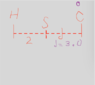# Displacement Problem: Sandra Walks Home from C

• ValerieL
In summary, Sandra walks home from the commercial mall. Her displacement is -2.5 if the origin is at the commercial mall, and -3 if the origin is at home.f

## Homework Statement

H------S-----C

Sandra is represented by S, Home is represented by H, and C is represented by commercial mall.
If Sandra walks home, what is her displacement if the commercial mall is the origin?

The distance between H and S is 2 miles and the distance between S and C is represented by D.
D = 3.0 miles.

## Homework Equations

Displacement = xf - xo/t

## The Attempt at a Solution

I'm not sure if I have the concept of displacement down right...it should be the right overall change in position from its start to final, but when I do this problem it doesn't make sense.

So C is the origin (xo) so its value is 5 and H is 0. So the overall displacement should be -5, but when I did the problem, it said -5 was incorrect. So I'm not sure what I did wrong.

Last edited by a moderator:
I think you assumed Sandra was at the mall.

If Sandra is in the center and the origin was the mall, then mall = x0. If she walks home, she is walking from the origin to home, right? So shouldn't that be -5? Even if she started from the center, it should be -3 or something?

##x_f## is the final position of Sandra and ##x_0## is the initial position of Sandra (not of the origin). The origin is needed in order to properly determine the values of ##x_f## and ##x_0##.
So if the origin is at C, what are the values of ##x_f## and ##x_0##? (I repeat again they are the final and initial values for the position of Sandra).
If the origin is at H, what are the values for ##x_f## and ##x_0##? As we change the origin, those values might be changing as well.

Seems to me that you have some misunderstanding as to what the origin is truly. The origin is the origin of the coordinate system we use, to determine the values for the positional coordinates (##x_f## and ##x_0##), it is not the starting place from which Sandra is starting walking.

Oh, that makes sense, that may be the case. Usually when you hear origin, you think that it is the starting place. Well, in that case, how would you be able to calculate the position of Sandra? If she goes to her home, then it is a negative displacement.

The distance between S and H is 2 and if the origin is at C, then we count C as 0 so S's location becomes 2.5 which is in between H (2 miles from Sandra) and C (3 miles from Sandra), so the displacement would be -2.5?

Looking at the picture, we have now marked the origin as C, does that mean S is at 3.0 right now or if 3.0 + 2 = 5 and she's in the middle, does it mean she's at 2.5. So then the distance between her the house is 2. but the house's value would be 5. xf=5 x0 = 3 or 2.5 so the displacement should be 2.5 or 3. Is this logic sound?

#### Attachments

•problem.png
11.1 KB · Views: 277
If we take C as the origin then for Sandra's final position we have ##x_f=5## and for Sandra's starting position we have ##x_0=3##, so the displacement is ##x_f-x_0=5-3=2miles##.

I don't understand your logic that S's location is 2.5, Sandra is not in the middle, Sandra's initial position is 3 miles from point C so ##x_0=3## .

•ValerieL
Oh okay, so we can't assume that S is 2.5 even if it looks like it is in center between H and C. So if the total distance is 5, we can't just divide it by 2 and say that S is at 2.5?

It is 2 miles distance but even if it would be 4-5 in terms of C being 0, would it also be considered negative displacement just because it is moving leftwards from S to the house?

No we can't assume that S is in position 2.5 miles from C , because the problem states clearly that Sandra is 3miles from the C.

To have negative displacement it must be ##x_f<x_0## . The positional coordinates ##x_f## and ##x_0## can be negative if we take as positive direction the direction to the right, so in that case it would have been ##x_f=-5##, ##x_0=-3##, hence the displacement would have been ##x_f-x_0=-5-(-3)=-2## also negative.